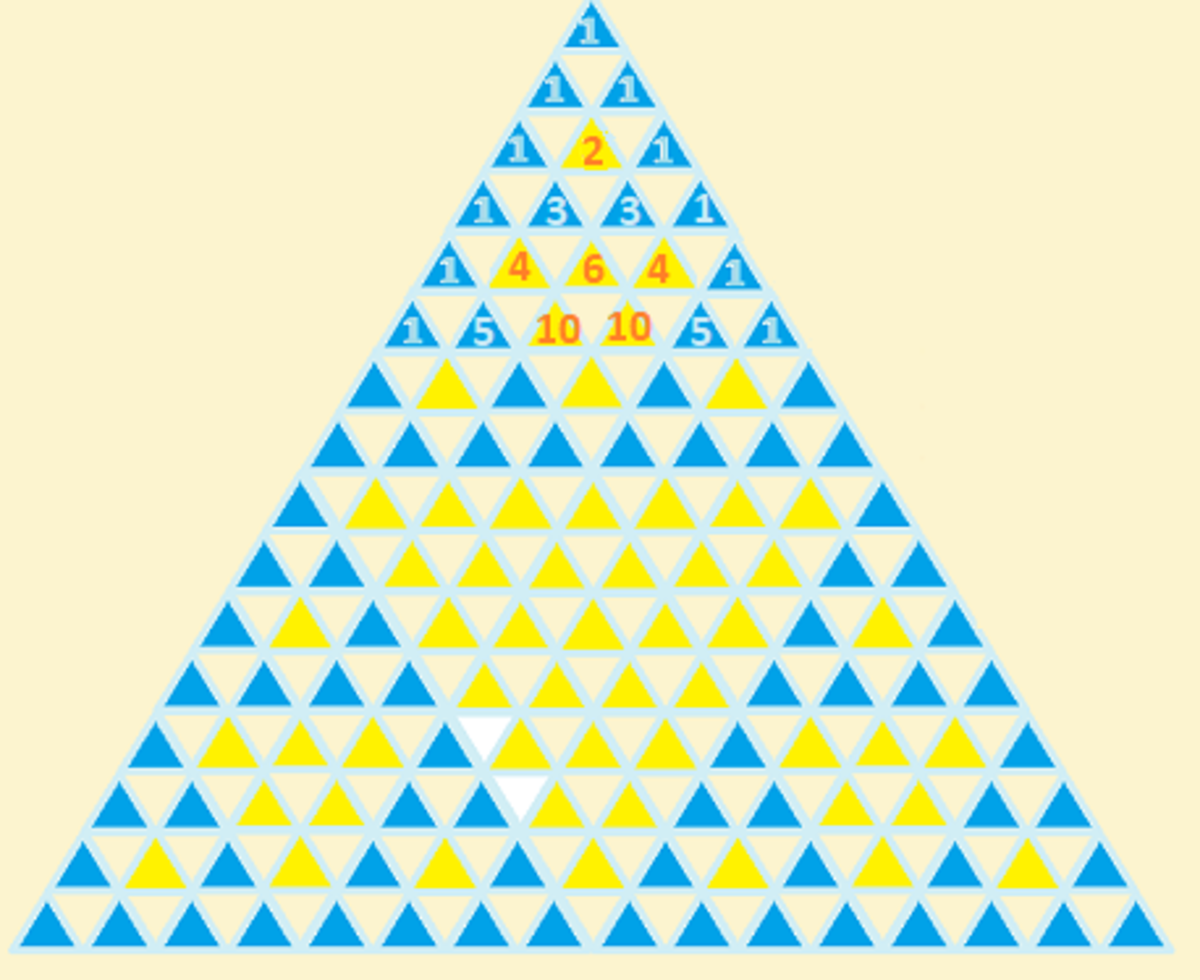# Interesting Facts About Pascal's Triangle

• Author:
• Updated date:

I am a former maths teacher and owner of DoingMaths. I love writing about maths, its applications, and fun mathematical facts.

## What Is Pascal's Triangle?

Pascal's Triangle is a number triangle that, although very easy to construct, has many interesting patterns and useful properties.

Although we name it after the French mathematician Blaise Pascal (1623–1662) who studied and published work on it, Pascal's Triangle is known to have been studied by the Persians during the 12th century, the Chinese during the 13th century and several 16th-century European mathematicians.

The Triangle's construction is very simple. Start with a 1 at the top. Each number below this is formed by adding together the two numbers diagonally above it (treating empty space on the edges as zero). Therefore the second row is 0 + 1 = 1 and 1 + 0 =1; the third row is 0 + 1 =1, 1 + 1 = 2, 1 + 0 =1 and so on.

## Hidden Number Patterns in Pascal's Triangle

If we look at the diagonals of Pascal's Triangle, we can see some interesting patterns. The outside diagonals consist entirely of 1s. If we consider that each end number will always have a 1 and a blank space above it, it is easy to see why this happens.

The second diagonal is the natural numbers in order (1, 2, 3, 4, 5, ...). Again, by following the construction pattern of the triangle, it is easy to see why this happens.

The third diagonal is where it gets really interesting. We have the numbers 1, 3, 6, 10, 15, 21, .... These are known as the triangle numbers, so-called as these numbers of counters can be arranged into equilateral triangles.

The triangle numbers are formed by each time adding one more than was added the previous time. So for example, we start with one, then we add two, then add three, then add four and so on giving us the sequence.

The fourth diagonal (1, 4, 10, 20, 35, 56, ...) is the tetrahedral numbers. These are similar to the triangle numbers, but this time forming 3-D triangles (tetrahedrons). These numbers are formed by adding consecutive triangle numbers each time, i.e. 1, 1 + 3 = 4, 4 + 6 = 10, 10 + 10 = 20, 20 + 15 = 35, etc.

The fifth diagonal (1, 5, 15, 35, 70, 126, ...) contains the pentatope numbers.

Scroll to Continue

## Binomial Expansions

Pascal's Triangle is also very useful when dealing with binomial expansions.

Consider (x + y) raised to consecutive whole number powers.

(x + y)1 = x + y

(x + y)2 = x2 + 2xy + y2

(x + y)3 = x3 + 3x2y + 3xy2 + y3

(x + y)4 = x4 + 4x3y + 6x2y2 + 4xy3 + y4 etc.

The coefficients of each term match the rows of Pascal's Triangle. We can use this fact to quickly expand (x + y)n by comparing to the nth row of the triangle e.g. for (x + y)7 the coefficients must match the 7th row of the triangle (1, 7, 21, 35, 35, 21, 7, 1).

## The Fibonacci Sequence

Take a look at the diagram of Pascal's Triangle below. It is the usual triangle, but with parallel, oblique lines added to it which each cut through several numbers. Let's add together the numbers on each line:

• 1st line: 1
• 2nd line: 1
• 3rd line: 1 + 1 = 2
• 4th line: 1 + 2 = 3
• 5th line: 1 + 3 + 1 = 5
• 6th line: 1 + 4 + 3 = 8 etc.

By adding together the numbers on each line, we get the sequence: 1, 1, 2, 3, 5, 8, 13, 21, etc. otherwise known as the Fibonacci sequence (a sequence defined by adding the previous two numbers together to get the next number in the sequence).

## Patterns in Rows

There are also some interesting facts to be seen in the rows of Pascal's Triangle.

• If you sum all the numbers in a row, you will get twice the sum of the previous row e.g. 1, 1 + 1 = 2, 1 + 2 + 1 = 4, 1 + 3 + 3 + 1 = 8 etc. This is down to each number in a row being involved in the creation of two of the numbers below it.
• If the number of the row is prime (when counting rows, we say the top 1 is row zero, the pair of 1s is row one, and so on), then all of the numbers in that row (except for the 1s on the ends) are multiples of p. This can be seen in the 2nd, 3rd, 5th and 7th rows of our diagram above.

## Fractals in Pascal's Triangle

One amazing property of Pascal's Triangle becomes apparent if you colour in all of the odd numbers. Doing so reveals an approximation of the famous fractal known as Sierpinski's Triangle. The more rows of Pascal's Triangle that are used, the more iterations of the fractal are shown.The Sierpinski Triangle from Pascal's TriangleJacques Mrtzsn - https://commons.wikimedia.org/wiki/File:Pascal-Sierpinski.png

You can see in the image above that colouring in the odd numbers on the first 16 lines of Pascal's Triangle reveals the third step in constructing Sierpinski's Triangle.